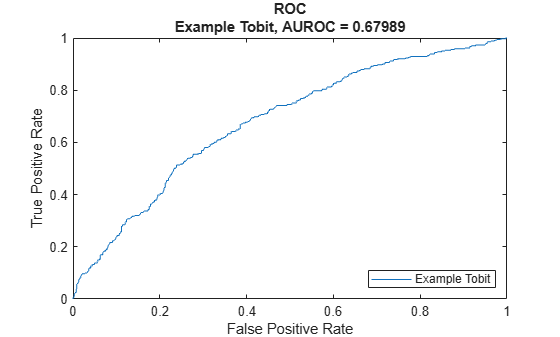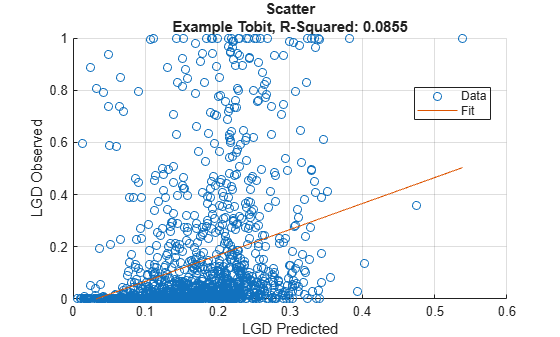# Tobit

Create `Tobit` model object for loss given default

Since R2021a

## Description

Create and analyze a `Tobit` model object to calculate loss given default (LGD) using this workflow:

1. Use `fitLGDModel` to create a `Tobit` model object.

2. Use `predict` to predict the LGD.

3. Use `modelDiscrimination` to return AUROC and ROC data. You can plot the results using `modelDiscriminationPlot`.

4. Use `modelCalibration` to return the R-squared, RMSE, correlation, and sample mean error of predicted and observed LGD data. You can plot the results using `modelCalibrationPlot`.

## Creation

### Syntax

``TobitLGDModel = fitLGDModel(data,ModelType)``
``TobitLGDModel = fitLGDModel(___,Name,Value)``

### Description

example

````TobitLGDModel = fitLGDModel(data,ModelType)` creates a `Tobit` LGD model object.```

example

````TobitLGDModel = fitLGDModel(___,Name,Value)` specifies options using one or more name-value pair arguments in addition to the input arguments in the previous syntax. The optional name-value pair arguments set the model object properties. For example, ```lgdModel = fitLGDModel(data,'tobit','PredictorVars',{'LTV' 'Age' 'Type'},'ResponseVar','LGD','CensoringSide','left','LeftLimit',1e-4)``` creates a `lgdModel` object using a `Tobit` model type. ```

### Input Arguments

expand all

Data for loss given default, specified as a table.

Data Types: `table`

Model type, specified as a string with the value of `"Tobit"` or a character vector with the value of `'Tobit'`.

Data Types: `char` | `string`

Name-Value Arguments

Specify optional pairs of arguments as `Name1=Value1,...,NameN=ValueN`, where `Name` is the argument name and `Value` is the corresponding value. Name-value arguments must appear after other arguments, but the order of the pairs does not matter.

Before R2021a, use commas to separate each name and value, and enclose `Name` in quotes.

Example: ```lgdModel = fitLGDModel(data,'tobit','PredictorVars',{'LTV' 'Age' 'Type'},'ResponseVar','LGD','CensoringSide','left','LeftLimit',1e-4)```

User-defined model ID, specified as the comma-separated pair consisting of `'ModelID'` and a string or character vector. The software uses the `ModelID` text to format outputs and is expected to be short.

Data Types: `string` | `char`

User-defined description for model, specified as the comma-separated pair consisting of `'Description'` and a string or character vector.

Data Types: `string` | `char`

Predictor variables, specified as the comma-separated pair consisting of `'PredictorVars'` and a string array or cell array of character vectors. `PredictorVars` indicates which columns in the `data` input contain the predictor information. By default, `PredictorVars` is set to all the columns in the `data` input except for `ResponseVar`.

Data Types: `string` | `cell`

Response variable, specified as the comma-separated pair consisting of `'ResponseVar'` and a string or character vector. The response variable contains the LGD data and must be a numeric variable. An LGD value of `0` indicates no loss (full recovery), `1` indicates total loss (no recovery), and values between `0` and `1` indicate a partial loss. By default, `ResponseVar` is set to the last column.

Data Types: `string` | `char`

Censoring side, specified as the comma-separated pair consisting of `'CensoringSide'` and a character vector or string. `CensoringSide` indicates whether the desired Tobit model is left-censored, right-censored, or censored on both sides.

Data Types: `string` | `char`

Left-censoring limit, specified as the comma-separated pair consisting of `'LeftLimit'` and a scalar numeric between `0` and `1`.

Data Types: `double`

Right-censoring limit, specified as the comma-separated pair consisting of `'RightLimit'` and a scalar numeric between `0` and `1`.

Data Types: `double`

Options for fitting, specified as the comma-separated pair consisting of `'SolverOptions'` and an `optimoptions` object that is created using `optimoptions` from Optimization Toolbox™. The defaults for the `optimoptions` object are:

• `"Display"``"none"`

• `"Algorithm"``"sqp"`

• `"MaxFunctionEvaluations"``500` ✕ Number of model coefficients

• `"MaxIterations"` — The number of Tobit model coefficients is determined at run time, it depends on the number of predictors and the number of categories in the categorical predictors.

Note

When using `optimoptions` with a Tobit model, specify the `SolverName` as `fmincon`.

Data Types: `object`

## Properties

expand all

User-defined model ID, returned as a string.

Data Types: `string`

User-defined description, returned as a string.

Data Types: `string`

Underlying statistical model, returned as a compact linear model object. The compact version of the underlying regression model is an instance of the `classreg.regr.CompactLinearModel` class. For more information, see `fitlm` and `CompactLinearModel`.

Data Types: `CompactLinearModel`

Predictor variables, returned as a string array.

Data Types: `string`

Response variable, returned as a string.

Data Types: `string`

Censoring side, returned as a string.

Data Types: `string`

Left-censoring limit, returned as a scalar numeric between `0` and `1`.

Data Types: `double`

Right-censoring limit, returned as a scalar numeric between `0` and `1`.

Data Types: `double`

## Object Functions

 `predict` Predict loss given default `modelDiscrimination` Compute AUROC and ROC data `modelDiscriminationPlot` Plot ROC curve `modelCalibration` Compute R-square, RMSE, correlation, and sample mean error of predicted and observed LGDs `modelCalibrationPlot` Scatter plot of predicted and observed LGDs

## Examples

collapse all

This example shows how to use `fitLGDModel` to create a `Tobit` model for loss given default (LGD).

```load LGDData.mat head(data)```
``` LTV Age Type LGD _______ _______ ___________ _________ 0.89101 0.39716 residential 0.032659 0.70176 2.0939 residential 0.43564 0.72078 2.7948 residential 0.0064766 0.37013 1.237 residential 0.007947 0.36492 2.5818 residential 0 0.796 1.5957 residential 0.14572 0.60203 1.1599 residential 0.025688 0.92005 0.50253 investment 0.063182 ```
```rng('default'); NumObs = height(data); c = cvpartition(NumObs,'HoldOut',0.4); TrainingInd = training(c); TestInd = test(c);```

Create `Tobit` LGD Model

Use `fitLGDModel` to create a `Tobit` model using the `TrainingInd` data.

```lgdModel = fitLGDModel(data(TrainingInd,:),'Tobit',... 'ModelID','Example Tobit',... 'PredictorVars',{'LTV' 'Age' 'Type'},... 'ResponseVar','LGD',... 'CensoringSide','left',... 'LeftLimit',1e-4); disp(lgdModel)```
``` Tobit with properties: CensoringSide: "left" LeftLimit: 1.0000e-04 RightLimit: 1 ModelID: "Example Tobit" Description: "" UnderlyingModel: [1x1 risk.internal.credit.TobitModel] PredictorVars: ["LTV" "Age" "Type"] ResponseVar: "LGD" ```

Display the underlying model. The underlying model is a left-censored Tobit model. Use the `'CensoringSide'` argument and the `'LeftLimit'` `and` `'RightLimit'` arguments to modify the underlying Tobit model.

`disp(lgdModel.UnderlyingModel)`
```Tobit regression model, left-censored: LGD = max(0.0001,Y*) Y* ~ 1 + LTV + Age + Type Estimated coefficients: Estimate SE tStat pValue ________ _________ ______ __________ (Intercept) 0.057356 0.026651 2.1521 0.031502 LTV 0.2003 0.030673 6.5301 8.2231e-11 Age -0.09405 0.0072966 -12.89 0 Type_investment 0.10071 0.01791 5.6233 2.1247e-08 (Sigma) 0.28833 0.0055229 52.205 0 Number of observations: 2093 Number of left-censored observations: 547 Number of uncensored observations: 1546 Number of right-censored observations: 0 Log-likelihood: -638.353 ```

Predict LGD

For Tobit models, use `predict` to calculate the predicted LGD value, which is the unconditional expected value of the response, given the predictor values.

`predictedLGD = predict(lgdModel,data(TestInd,:))`
```predictedLGD = 1394×1 0.0871 0.1228 0.3181 0.0926 0.1654 0.2215 0.2347 0.0102 0.1576 0.1969 ⋮ ```

Validate LGD Model

Use `modelDiscriminationPlot` to plot the ROC curve.

`modelDiscriminationPlot(lgdModel,data(TestInd,:))`Use `modelCalibrationPlot` to show a scatter plot of the predictions.

`modelCalibrationPlot(lgdModel,data(TestInd,:))`